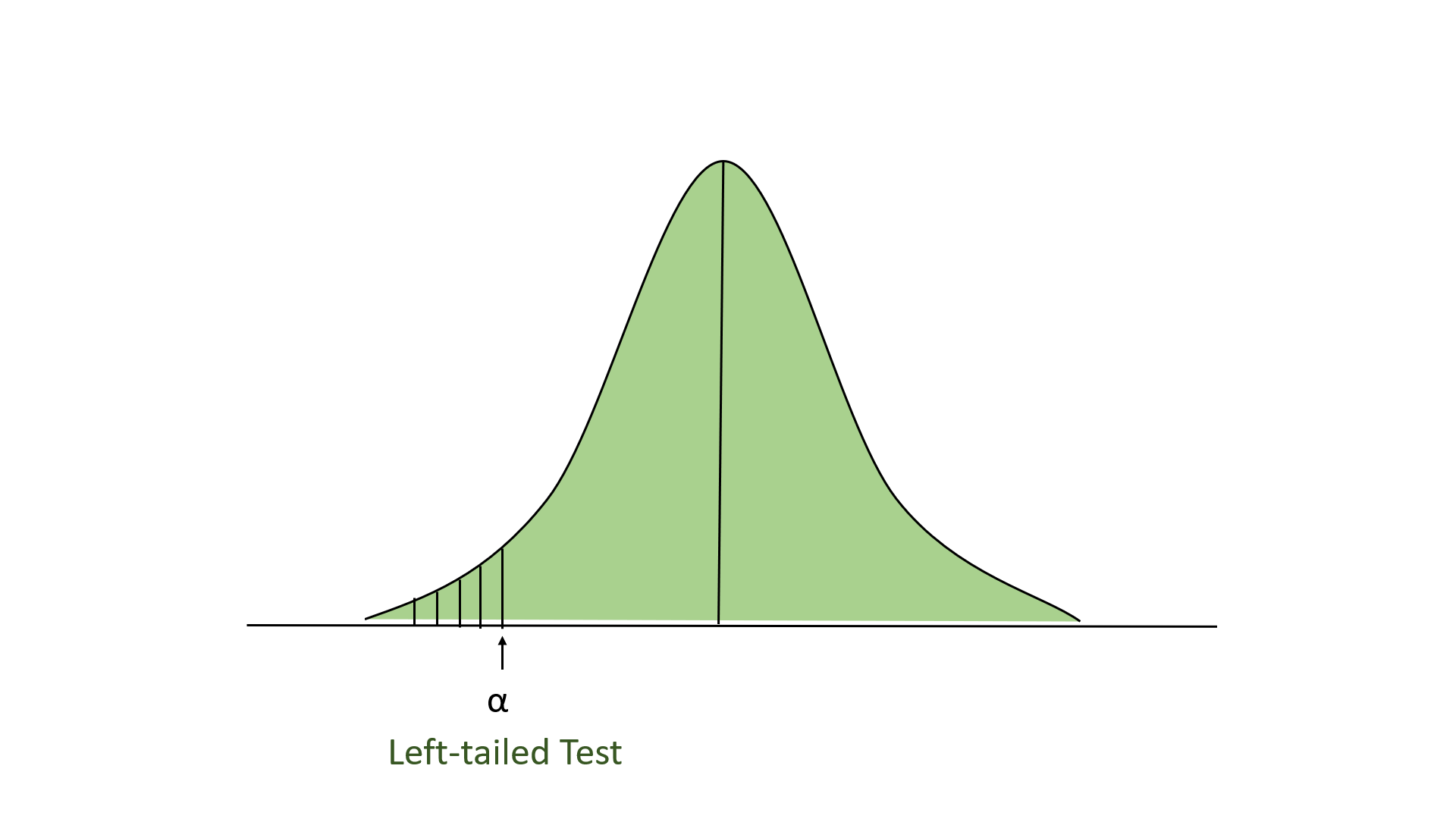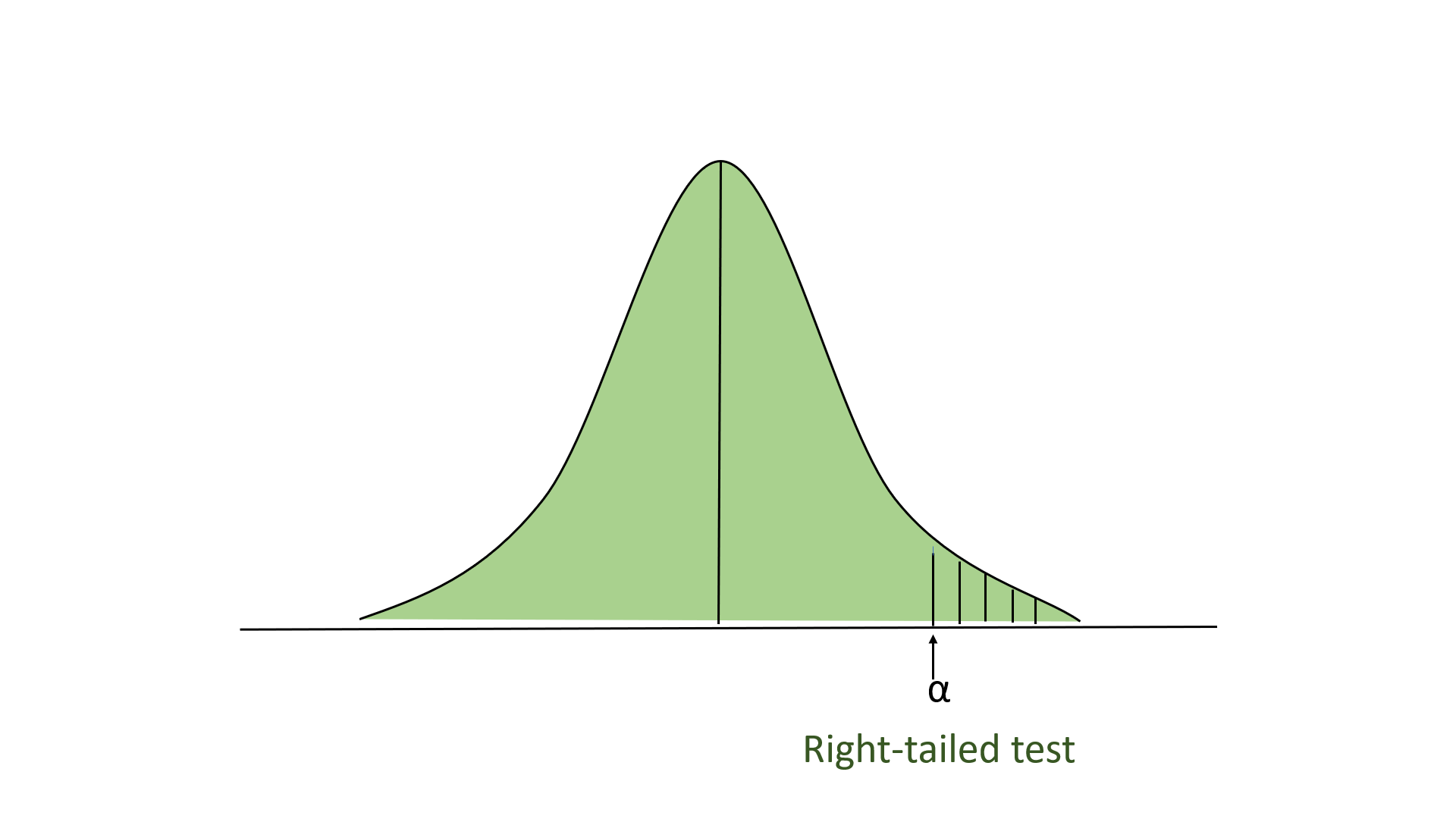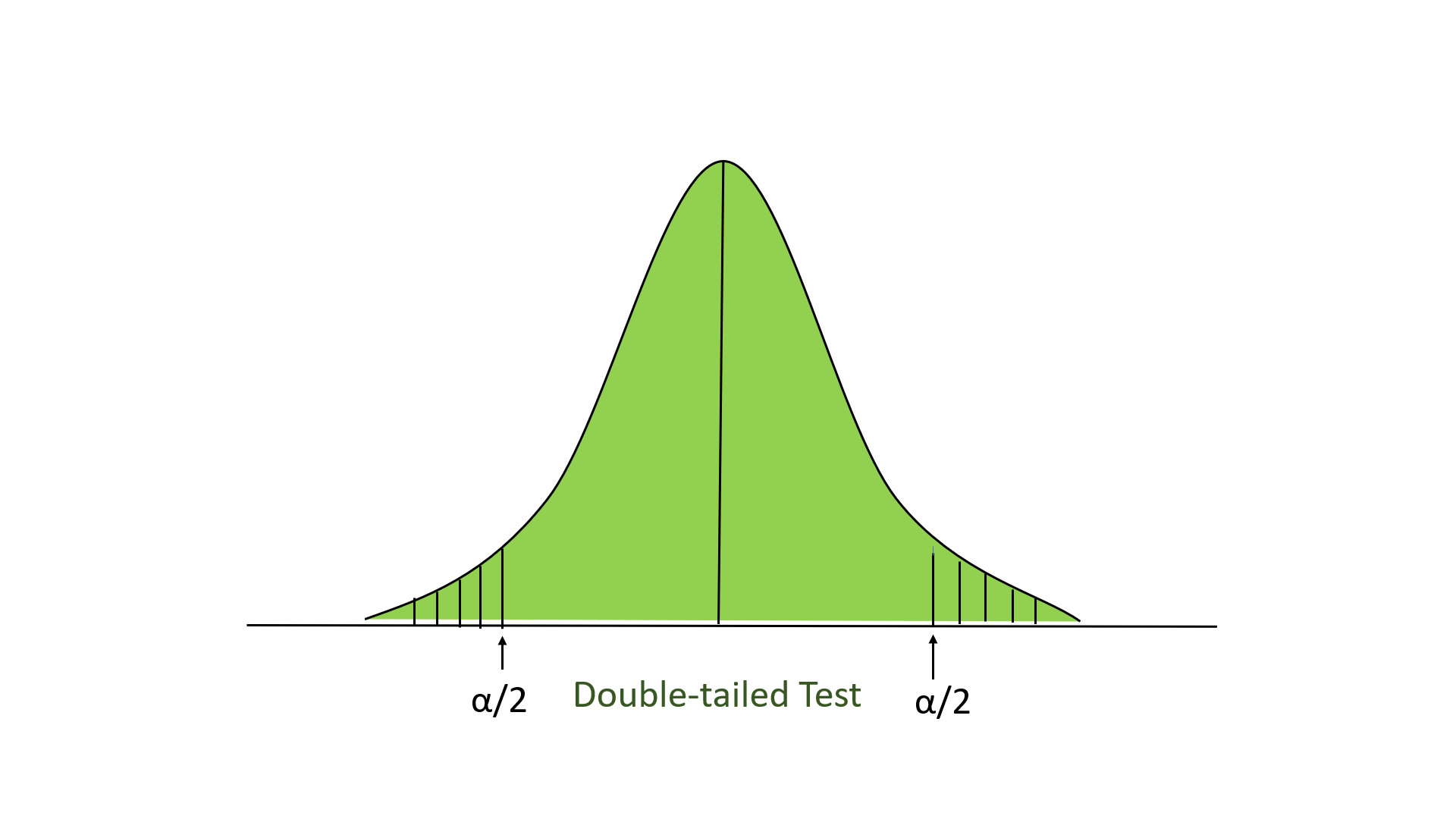Open in App
Not now

# Z-test

• Difficulty Level : Easy
• Last Updated : 20 Jul, 2021

Z-test is a statistical method to determine whether the distribution of the test statistics can be approximated by a normal distribution. It is the method to determine whether two sample means are approximately the same or different when their variance is known and the sample size is large (should be >= 30).

When to Use Z-test:

• The sample size should be greater than 30. Otherwise, we should use the t-test.
• Samples should be drawn at random from the population.
• The standard deviation of the population should be known.
• Samples that are drawn from the population should be independent of each other.
• The data should be normally distributed, however for large sample size, it is assumed to have a normal distribution.

Hypothesis Testing

A hypothesis is an educated guess/claim about a particular property of an object. Hypothesis testing is a way to validate the claim of an experiment.

• Null Hypothesis: The null hypothesis is a statement that the value of a population parameter (such as proportion, mean, or standard deviation) is equal to some claimed value. We either reject or fail to reject the null hypothesis. Null Hypothesis is denoted by H0.
• Alternate Hypothesis: The alternative hypothesis is the statement that the parameter has a value that is different from the claimed value. It is denoted by HA.

Level of significance: It means the degree of significance in which we accept or reject the null-hypothesis. Since in most of the experiments 100% accuracy is not possible for accepting or rejecting a hypothesis, so we, therefore, select a level of significance. It is denoted by alpha (∝).

Steps to perform Z-test:

• First, identify the null and alternate hypotheses.
• Determine the level of significance (∝).
• Find the critical value of z in the z-test using
• Calculate the z-test statistics. Below is the formula for calculating the z-test statistics.• where,
• X¯: mean of the sample.
• Mu: mean of the population.
• Sd: Standard deviation of the population.
• n: sample size.
• Now compare with the hypothesis and decide whether to reject or not to reject the null hypothesis

Type of Z-test

• Left-tailed Test: In this test, our region of rejection is located to the extreme left of the distribution. Here our null hypothesis is that the claimed value is less than or equal to the mean population value.• Right-tailed Test: In this test, our region of rejection is located to the extreme right of the distribution. Here our null hypothesis is that the claimed value is less than or equal to the mean population value.• Two-tailed test: In this test, our region of rejection is located to both extremes of the distribution. Here our null hypothesis is that the claimed value is equal to the mean population value.Below is the example of performing the z-test:

Problem: A school claimed that the students’ study that is more intelligent than the average school. On calculating the IQ scores of 50 students, the average turns out to be 11. The mean of the population IQ is 100 and the standard deviation is 15. State whether the claim of principal is right or not at a 5% significance level.

• First, we define the null hypothesis and the alternate hypothesis. Our null hypothesis will be:and our alternate hypothesis.• State the level of significance. Here, our level of significance given in this question (∝ =0.05), if not given then we take ∝=0.05.
• Now, we look up to the z-table. For the value of ∝=0.05, the z-score for the right-tailed test is 1.645.
• Now, we perform the Z-test on the problem:• Where:
• X = 110
• Mean (mu) = 100
• Standard deviation (sigma) = 15
• Significance level (alpha) = 0.05
• n = 50• Here 4.71 >1.645, so we reject the null hypothesis. If z-test statistics is less than z-score, then we will not reject the null hypothesis.

## Python3

 # importsimport mathimport numpy as npfrom numpy.random import randnfrom statsmodels.stats.weightstats import ztest  # Generate a random array of 50 numbers having mean 110 and sd 15# similar to the IQ scores data we assume abovemean_iq = 110sd_iq = 15/math.sqrt(50)alpha =0.05null_mean =100data = sd_iq*randn(50)+mean_iq# print mean and sdprint('mean=%.2f stdv=%.2f' % (np.mean(data), np.std(data)))  # now we perform the test. In this function, we passed data, in the value parameter# we passed mean value in the null hypothesis, in alternative hypothesis we check whether the# mean is larger  ztest_Score, p_value= ztest(data,value = null_mean, alternative='larger')# the function outputs a p_value and z-score corresponding to that value, we compare the # p-value with alpha, if it is greater than alpha then we do not null hypothesis # else we reject it.  if(p_value <  alpha):  print("Reject Null Hypothesis")else:  print("Fail to Reject NUll Hypothesis")

Reject Null Hypothesis


Two-sampled z-test:

In this test, we have provided 2 normally distributed and independent populations, and we have drawn samples at random from both populations. Here, we consider u1 and u2 be the population mean X1 and X2 are the observed sample mean. Here, our null hypothesis could be like:and alternative hypothesisand the formula for calculating the z-test score:where sigma1 and sigma2 are the standard deviation and n1 and n2 are the sample size of population corresponding to u1 and u2 .

Type 1 error and Type II error:

• Type I error: Type 1 error has occurred when we reject the null hypothesis, even when the hypothesis is true. This error is denoted by alpha.
• Type II error: Type II error has occurred when we didn’t reject the null hypothesis, even when the hypothesis is false. This error is denoted by beta.

My Personal Notes arrow_drop_up